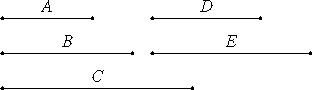# Proposition 28

To find medial straight lines commensurable in square only which contain a medial rectangle.

Set out the rational straight lines A, B, and C commensurable in square only. Take a mean proportional D between A and B. Let it be contrived that B is to C as D is to E.Since A and B are rational straight lines commensurable in square only, therefore the rectangle A by B, that is, the square on D, is medial. Therefore D is medial.

X.11

And since B and C are commensurable in square only, and B is to C as D is to E, therefore D and E are also commensurable in square only.

X.23,Note

But D is medial, therefore E is also medial.

Therefore D and E are medial straight lines commensurable in square only.

I say next that they also contain a medial rectangle.

V.16

Since B is to C as D is to E, therefore, alternately, B is to D as C is to E.

VI.16

But B is to D as D is to A, therefore D is to A as C is to E. Therefore the rectangle A by C equals the rectangle D by E.

X.21

But the rectangle A by C is medial, therefore the rectangle D by E is also medial.

Therefore medial straight lines commensurable in square only have been found which contain a medial rectangle.

Q.E.D.

## Guide

There has been no construction given in Book X for the required lines A, B, and C. One example would be where their lengths are 1, √2, and √ 3.

Set A to have length 1, B length √b, an irrational number where b is rational, and C length √c, an irrational number where c is rational, with b/c and irrational number. Then D has length b1/4 while E has length (√c)/b1/4. Then D and E are medial and commensurable in square only, and the b1/4 by (√c)/b1/4 rectangle they contain has area √c, an irrational number.

This proposition is used in X.75.